Connect with us

# Using Cash Models To Determine The Optimal Cash BalancePublished

on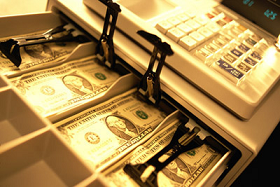Without monitoring your cash—how to measure it, invest it, borrow it, and collect it—you can cheat yourself out of extra profits or even avoid trouble with creditors and bankruptcy. The main objective of cash management is an optimal cash balance; minimizing the sum of fixed cost of transactions and the opportunity cost of holding cash balance.  Optimal balance here means a position when the cash balance amount is on the most ideal proportion so that the company has the ability to invest the excess cash for a return [profit] and at the same time have sufficient liquidity for future needs

The key ingredient here is that the cash balance should neither be excessive nor deficient. For example, companies with many bank accounts may be accumulating excessive balances. Do you know how much cash you need, how much you have, where the cash is, what the sources of cash are, and where the cash will be used? This is especially crucial in recession.

The minimum cash to hold is the greater of (1) compensating balances (a deposit held by a bank to compensate it for providing services) or (2) precautionary balances (money held in cash for an emergency) plus transaction balances (money to cover un cleared checks). The company needs sufficient cash to satisfy daily requirements. Determining the optimal cash balance is one among the most a crucial task in cash management area.

The question is; how to determine the optimal cash balance? William Baumol and Miller-Orr offer cash models to determine the optimal cash balance that you can use. Through this post I will introduce these two cash models and demonstrate how to use them with case examples.

### Determining Optimal Cash Balance Under Conditions of Certainty [William Baumol’s Cash Model]

William Baumol developed a cash model [see below exhibit] to determine the optimum amount of transaction cash under conditions of certainty.

The objective is to minimize the sum of the fixed costs of transactions and the opportunity cost of holding cash balances.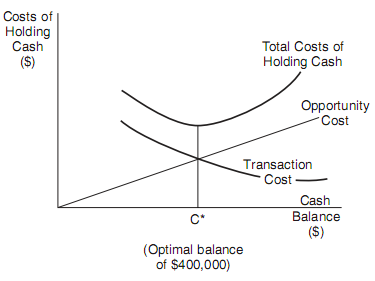These costs are expressed as:

F × [T/C] + i.[C/2]

Where:

F = the fixed cost of a transaction
T = the total cash needed for the time period involved
i = the interest rate on marketable securities
C = cash balance

The optimal level of cash is determined using the following formula: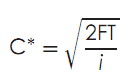Case Example

You estimate a cash need for \$4 million over a one month period where the cash account is expected to be disbursed at a constant rate. The opportunity interest rate is 6 percent per annum, or 0.5 percent for a one-month period. The transaction cost each time you borrow or withdraw is \$100.

The optimal transaction size (the optimal borrowing or withdrawal lot size) and the number of trans actions you should make during the month follow: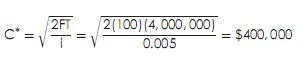• The optimal transaction size is: \$400,000.
• The average cash balance is: C/2 = \$400,000/2 = \$200,000
• The number of transactions required are:  \$4,000,000/\$400,000 = 10 transactions during the month.

### Determining Optimal Cash Balance Under Conditions of Uncertainty [Miller-Orr’s Cash Model]

You can use a stochastic model for cash management where uncertainty exists for cash payments. The Miller-Orr model places an upper and lower limit for cash balances. When the upper limit is reached, a transfer of cash to marketable securities is made. When the lower limit is reached, a transfer from securities to cash occurs. A transaction will not occur as long as the cash balance falls within the limits.

The Miller-Orr cash model takes into account the fixed costs of a securities transaction (F), which is assumed to be the same for buying as well as selling, the daily interest rate on marketable securities (i), and the variance of daily net cash flows [?2].

The purpose is to satisfy cash requirements at the least cost. A major assumption is the randomness of cash flows.

The two control limits in the Miller-Orr model may be specified as ‘‘d’’ dollars as an upper limit and zero dollars at the lower limit. When the cash balance reaches the upper level, d less z dollars of securities are bought and the new balance becomes z dollars. When the cash balance equals zero, z dollars of securities are sold and the new balance again reaches z. Of course, practically speaking, you should note that the minimum cash balance is established at an amount greater than zero due to delays in transfer as well as to having a safety buffer.

The optimal cash balance “z” is computed as: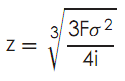The optimal value for d is computed as 3z. The average cash balance will approximate (z + d)/3

Case Example

You wish to use the Miller-Orr model. The following information is supplied:

• Fixed cost of a securities transaction = \$10
• Variance of daily net cash flows = \$50
• Daily interest rate on securities (10%/360) = 0.0003

The optimal cash balance, the upper limit of cash needed, and the average cash balance are: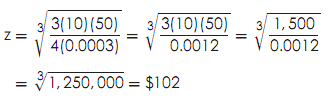The optimal cash balance is = \$102.

The upper limit is = \$306 [=3 × \$102].

The average cash balance is: \$136 = (\$102 + \$306)/3

A brief elaboration on these findings is needed for clarification. When the upper limit of \$306 is reached, \$204 of securities (\$306–\$102) will be purchased to bring you to the optimal cash balance of \$102.  When the lower limit of zero dollars is reached, \$102 of securities will be sold to again bring you to the optimal cash balance of \$102.

And the cash model is shown below: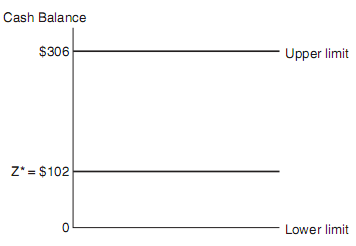Are you looking for easy accounting tutorial? Established since 2007, Accounting-Financial-Tax.com hosts more than 1300 articles (still growing), and has helped millions accounting student, teacher, junior accountants and small business owners, worldwide.